# RD Sharma Solutions for Class 11 Maths Chapter 31 Mathematical Reasoning

## RD Sharma Solutions Class 11 Maths Chapter 31 – Get Free PDF Updated for (2021-22)

RD Sharma Solutions for Class 11 Maths Chapter 31 – Mathematical Reasoning are provided here for students to score good marks in the board exams. RD Sharma Solutions for Class 11 Maths Chapter 31 Mathematical Reasoning are provided here for students to practice and prepare for their board exams. To facilitate easy learning and help students to understand the concepts explained in this chapter easily, RD Sharma Solutions are formulated here by our subject experts, as per the latest CBSE syllabus.

RD Sharma Class 11 Maths Solutions for Chapter Mathematical Reasoning can be downloaded in the form of a pdf and students can use it as a reference tool to quickly review all the topics. Students may face difficulty in answering all the exercise questions given in the textbook. The solved problems will help students prepare well and score good marks in the exam. Students are also suggested to solve previous year question papers and sample papers of Class 11 Maths, to gain that extra knowledge.

Chapter 31 – Mathematical Reasoning contains six exercises and the RD Sharma Solutions present in this page provide solutions to the questions present in each exercise. Now, let us have a look at the concepts discussed in this chapter.

• Statements.
• Negation of a statement.
• Compound statements.
• Basic connectives.
• The word ‘AND’.
• The word ‘OR’.
• Quantifiers.
• Implications.
• Validity of statements.
• Validity of statements with ‘AND’.
• Validity of statements with ‘OR’.
• Validity of statements with ‘IF-THEN’.
• Validity of statements with ‘IF AND ONLY IF’.
• Validity of statements by contradiction.
• Invalidity of statements by counter examples.

## Download the pdf of RD Sharma Solutions for Class 11 Maths Chapter 31 – Mathematical Reasoning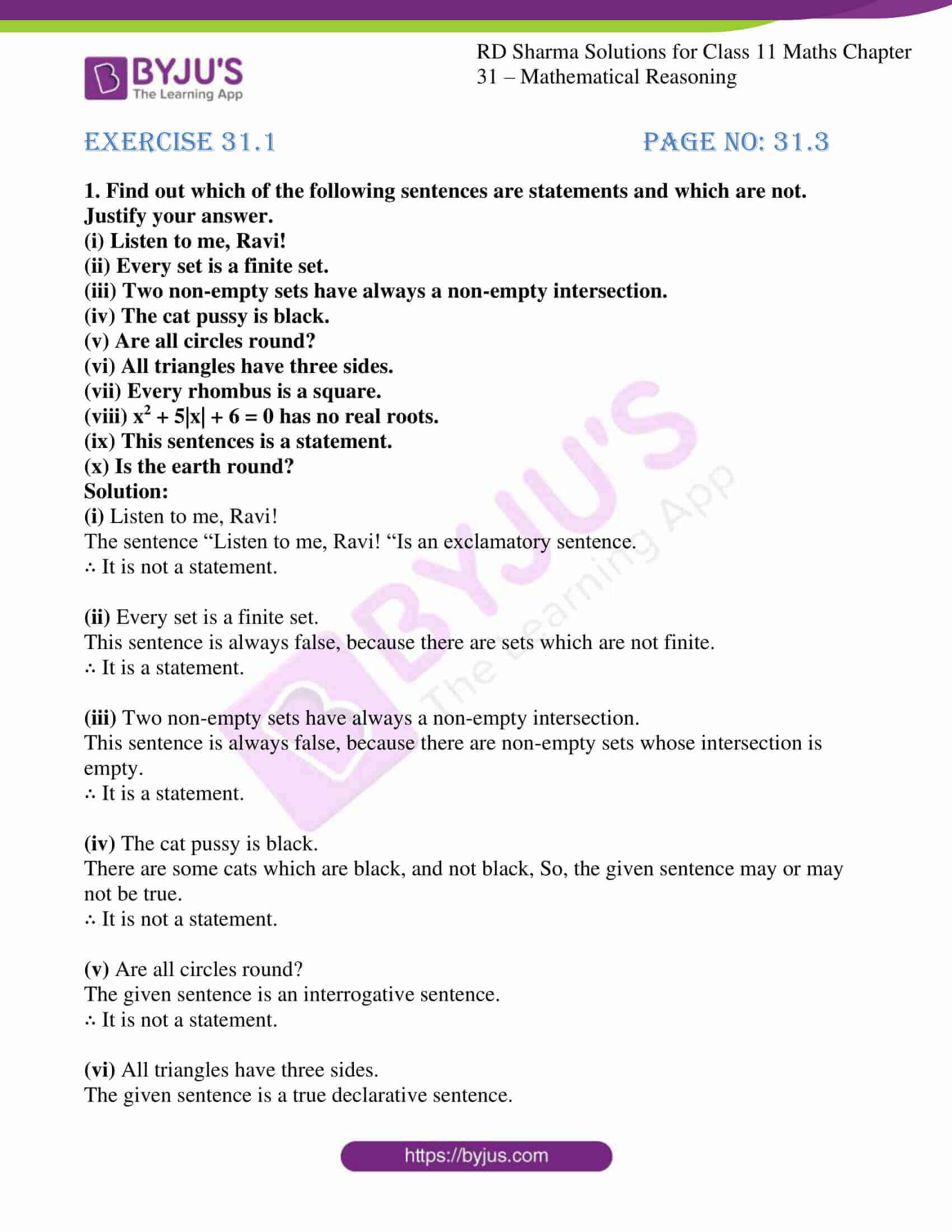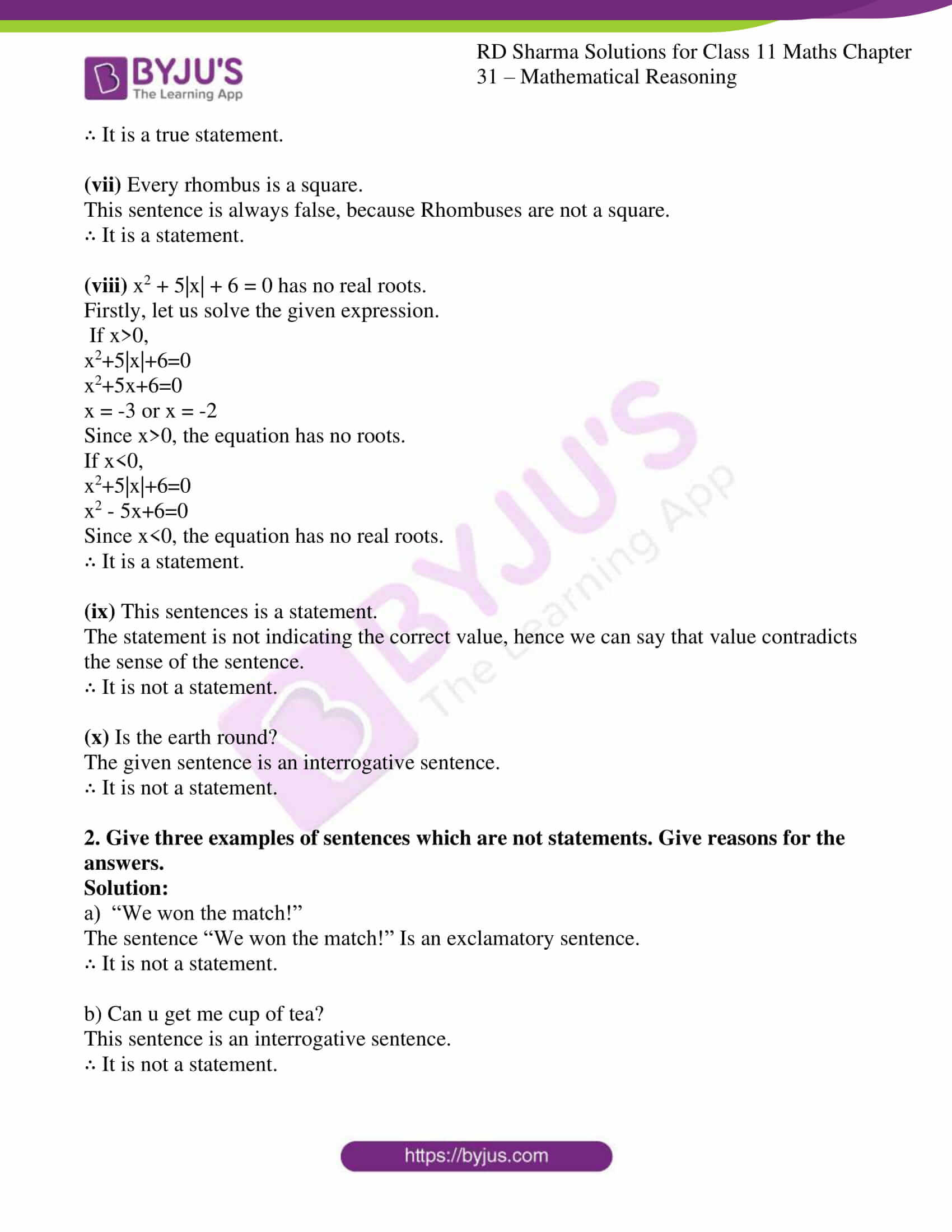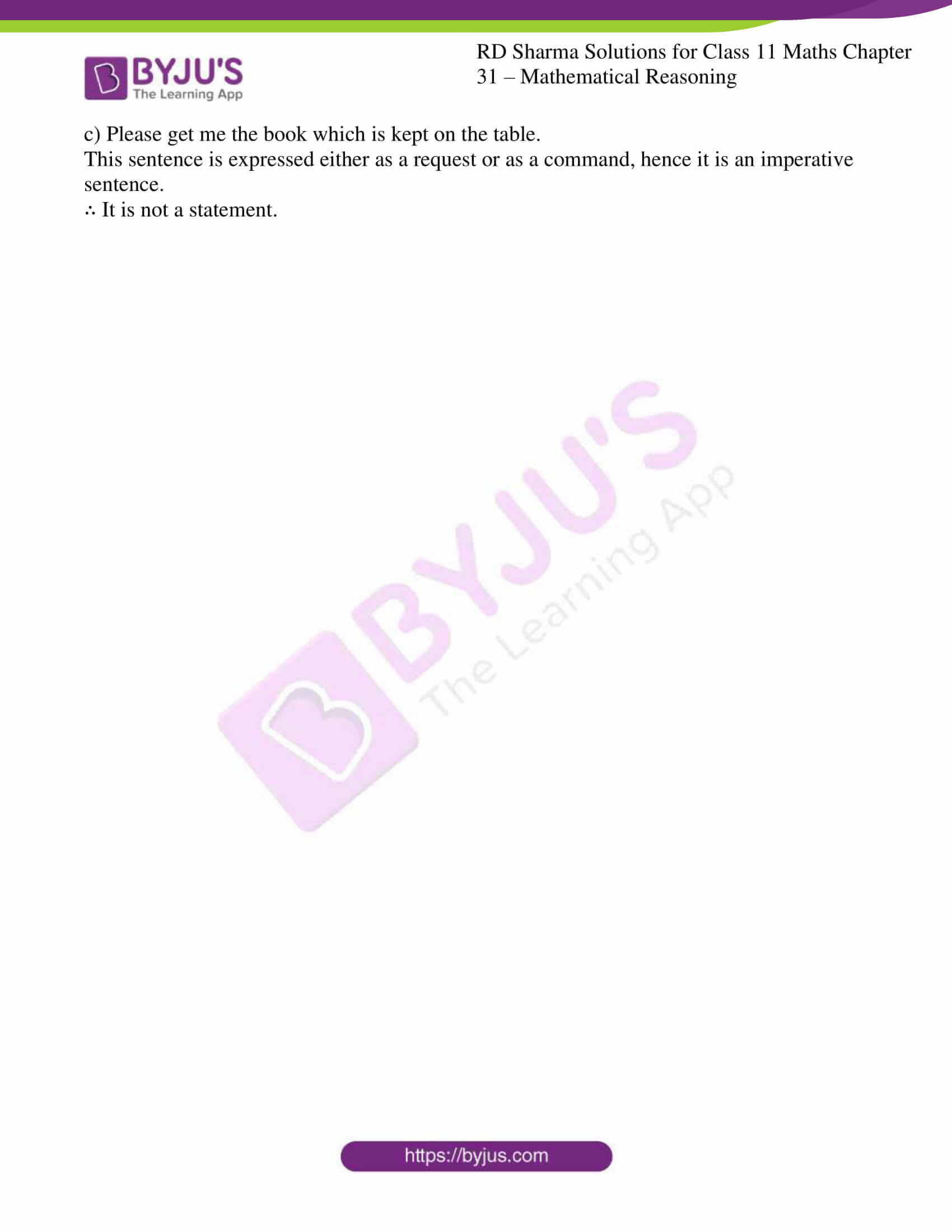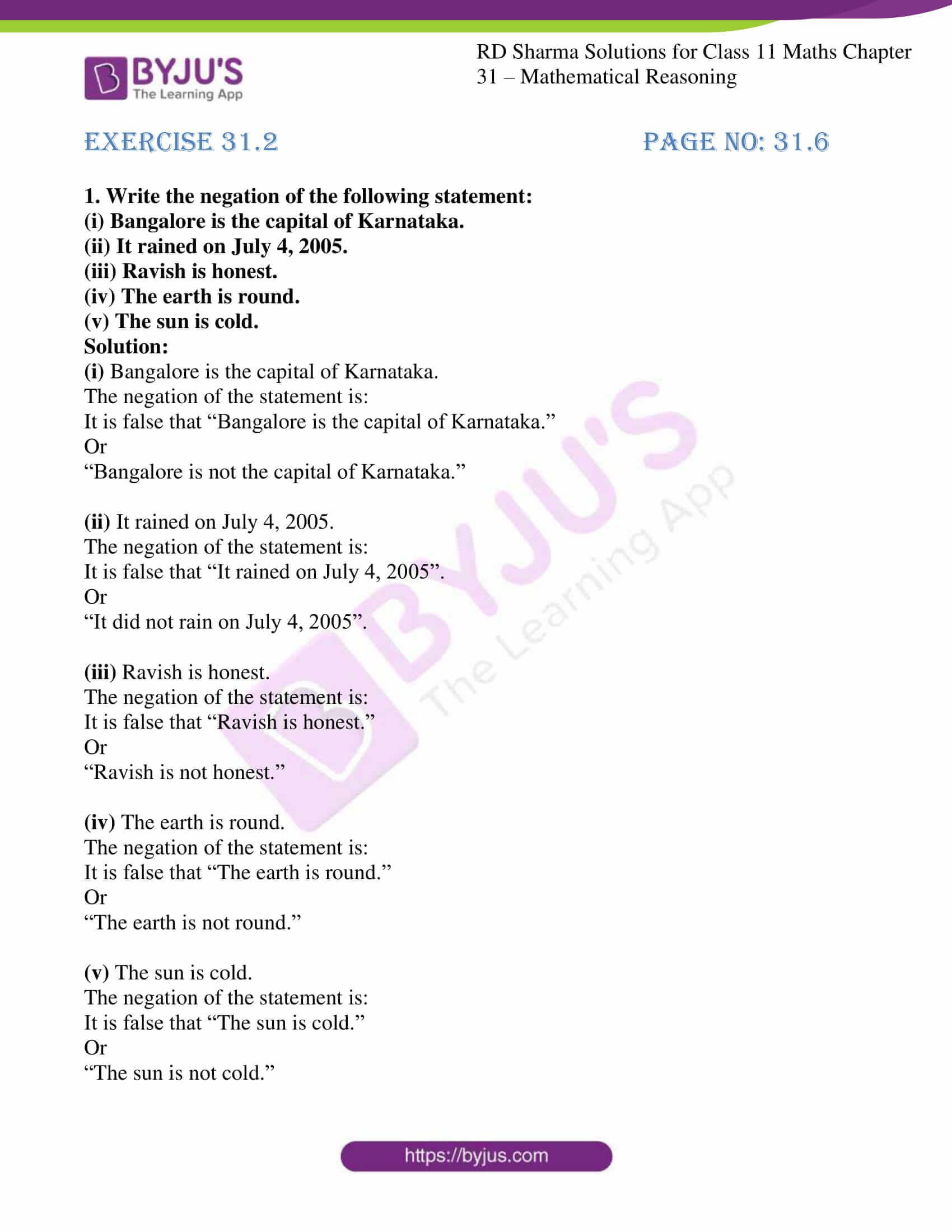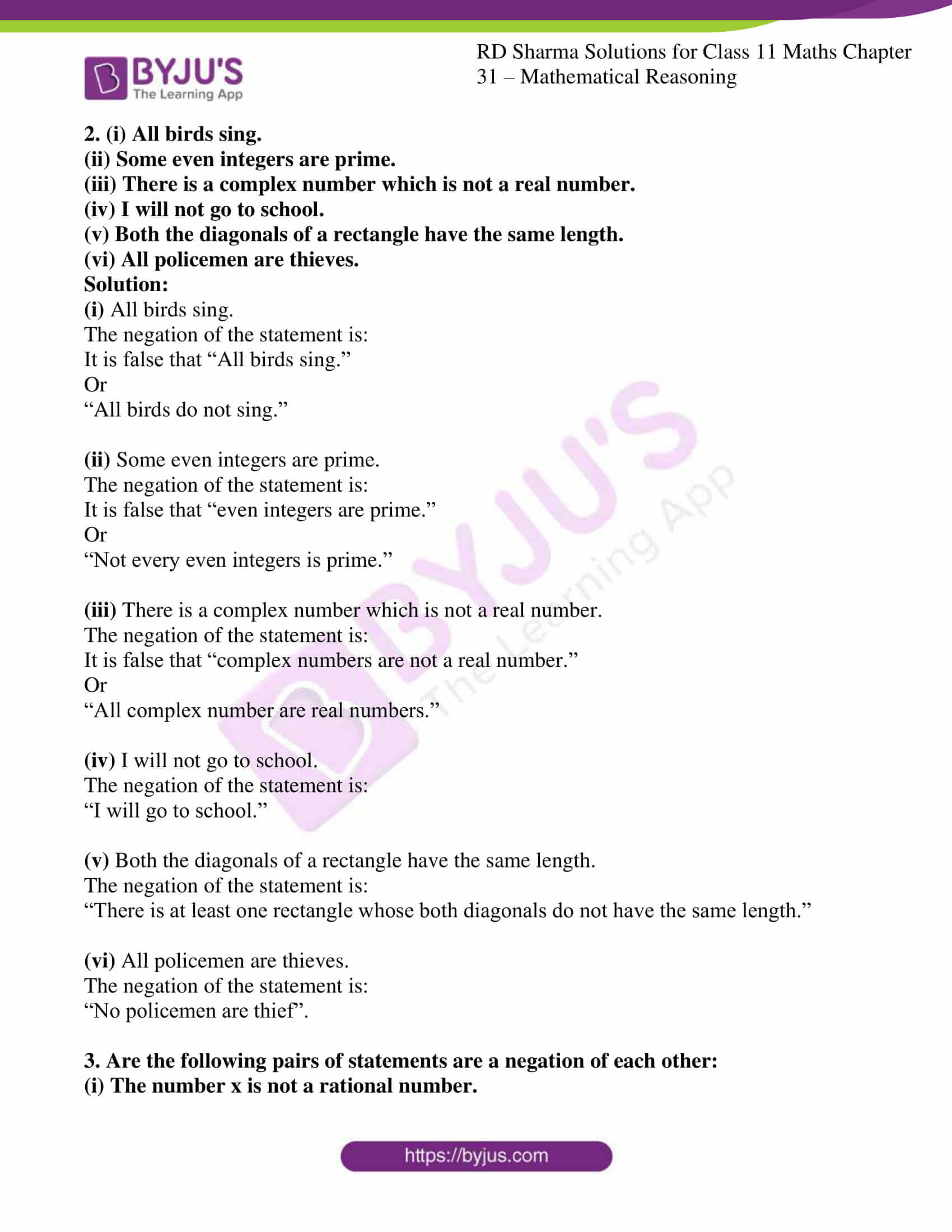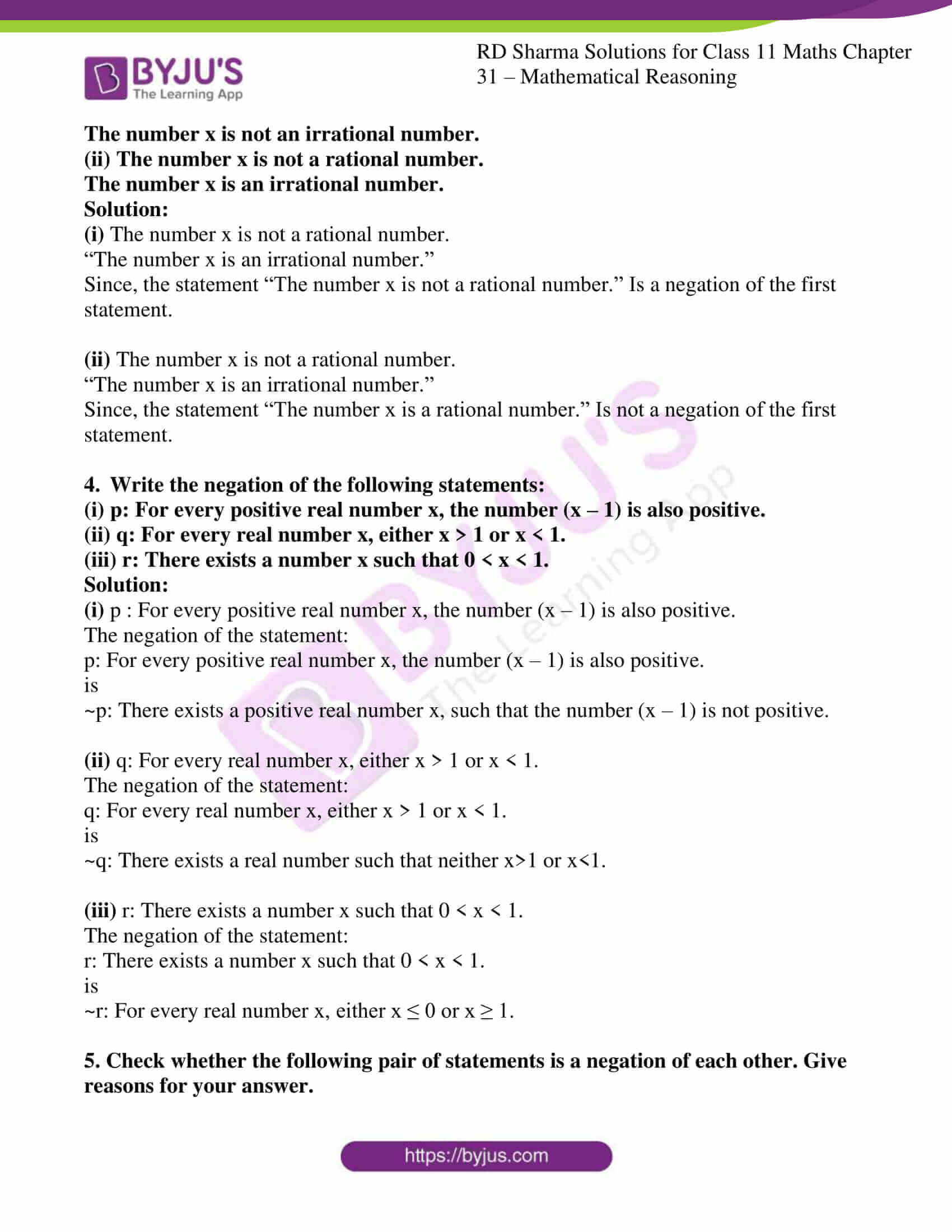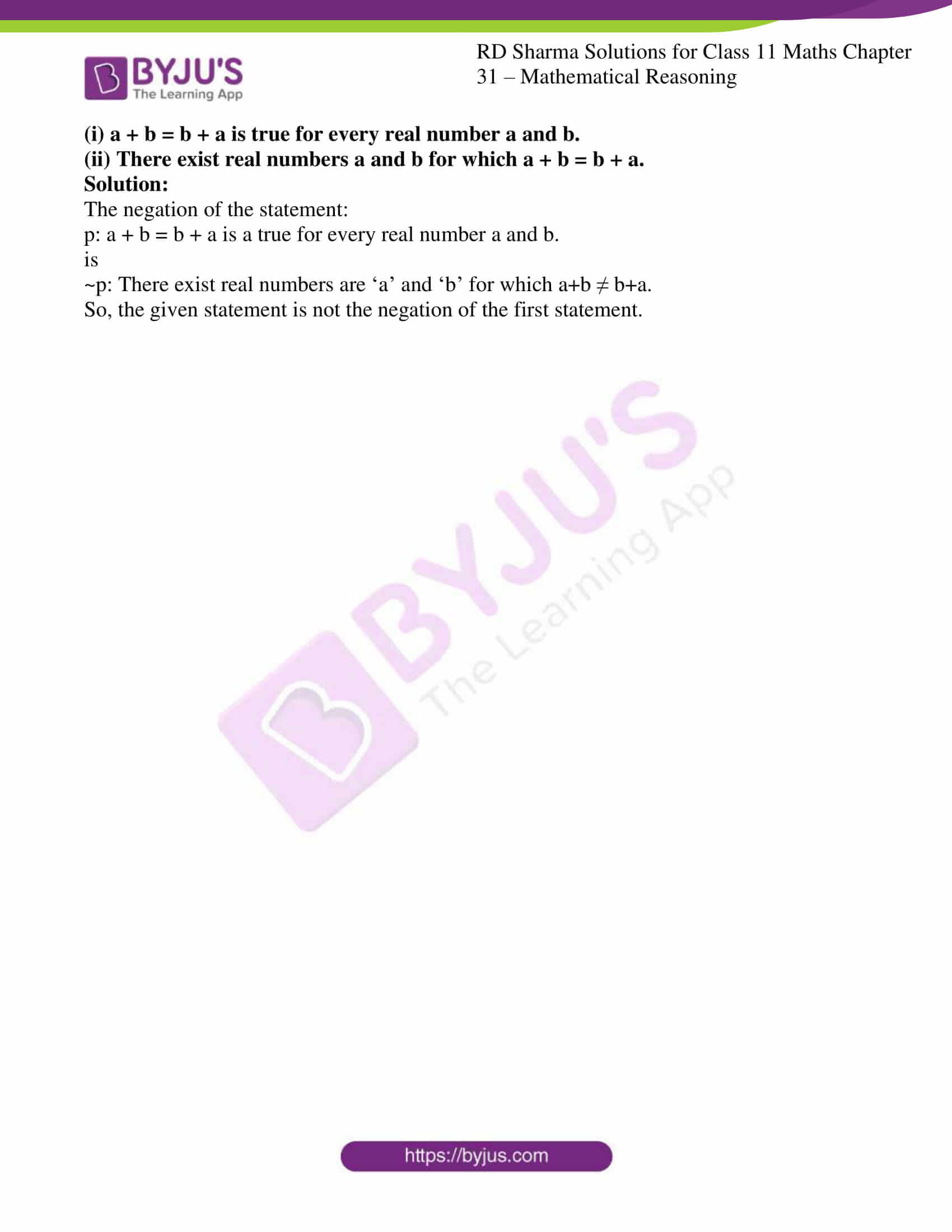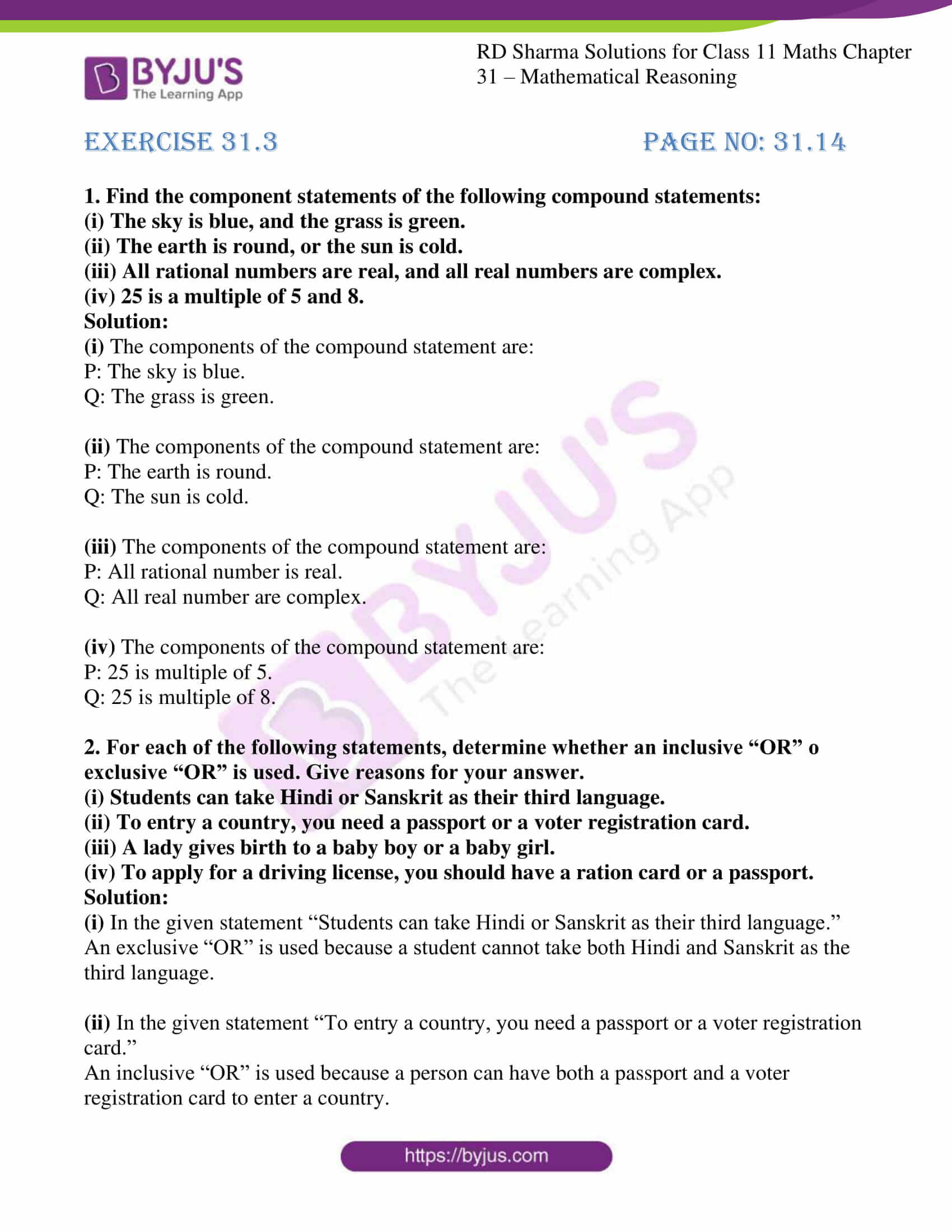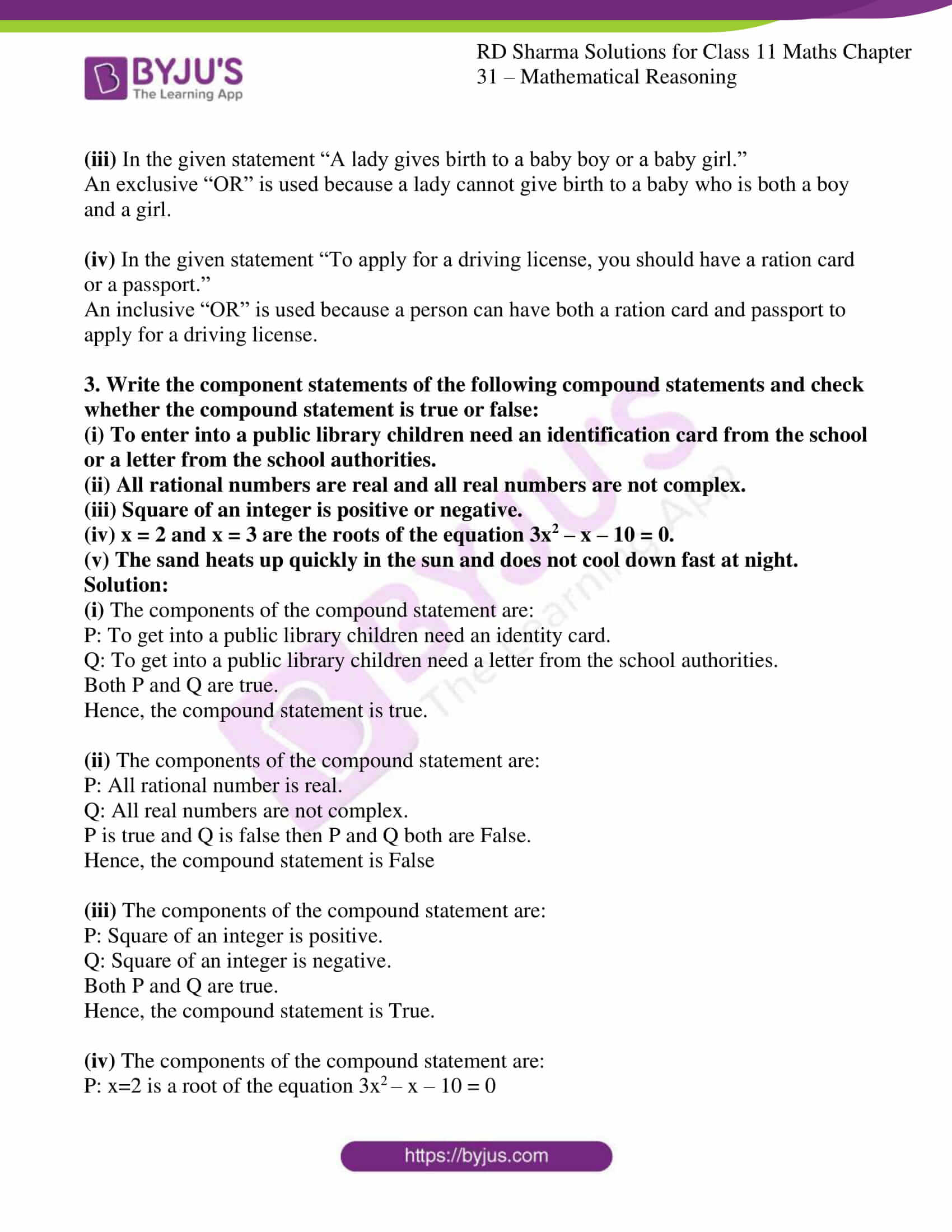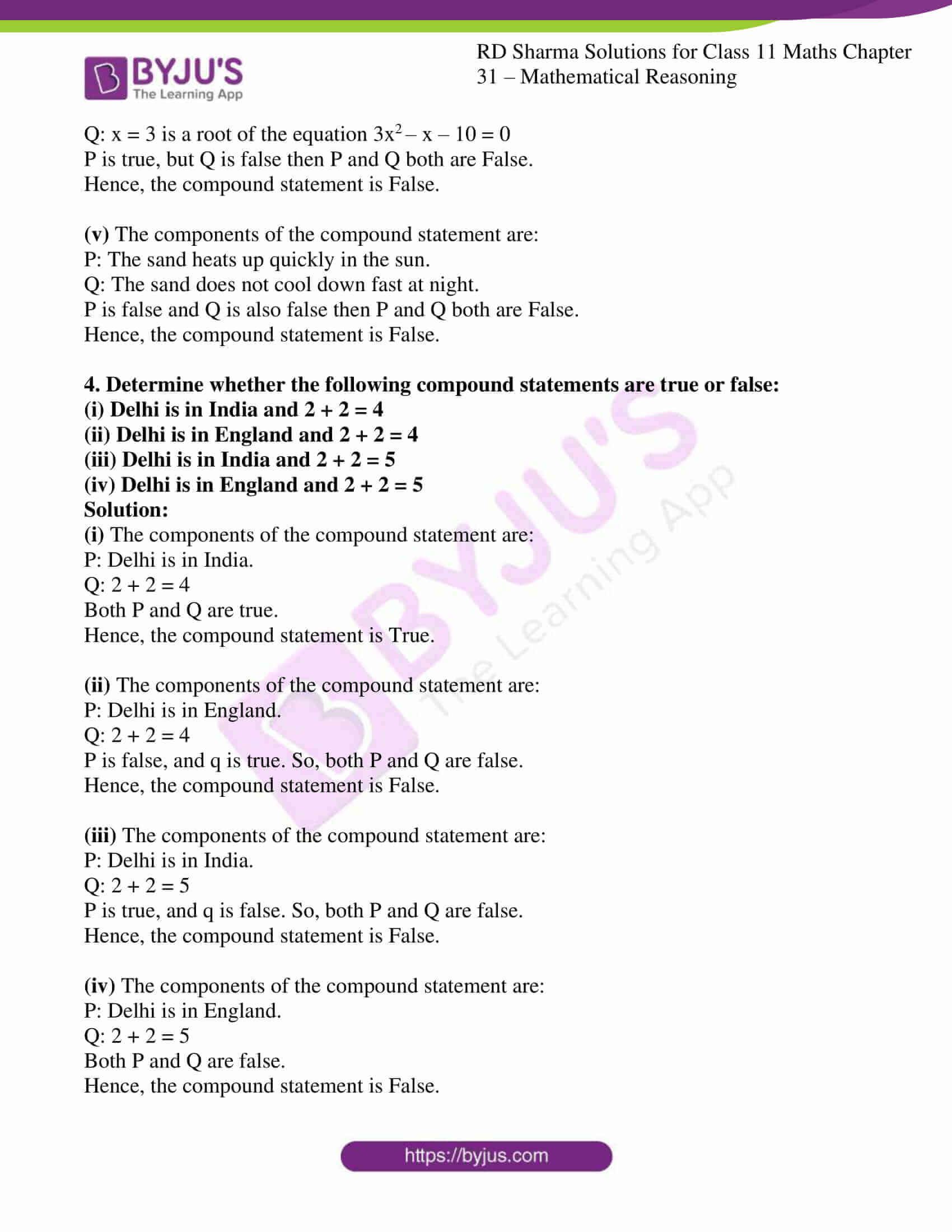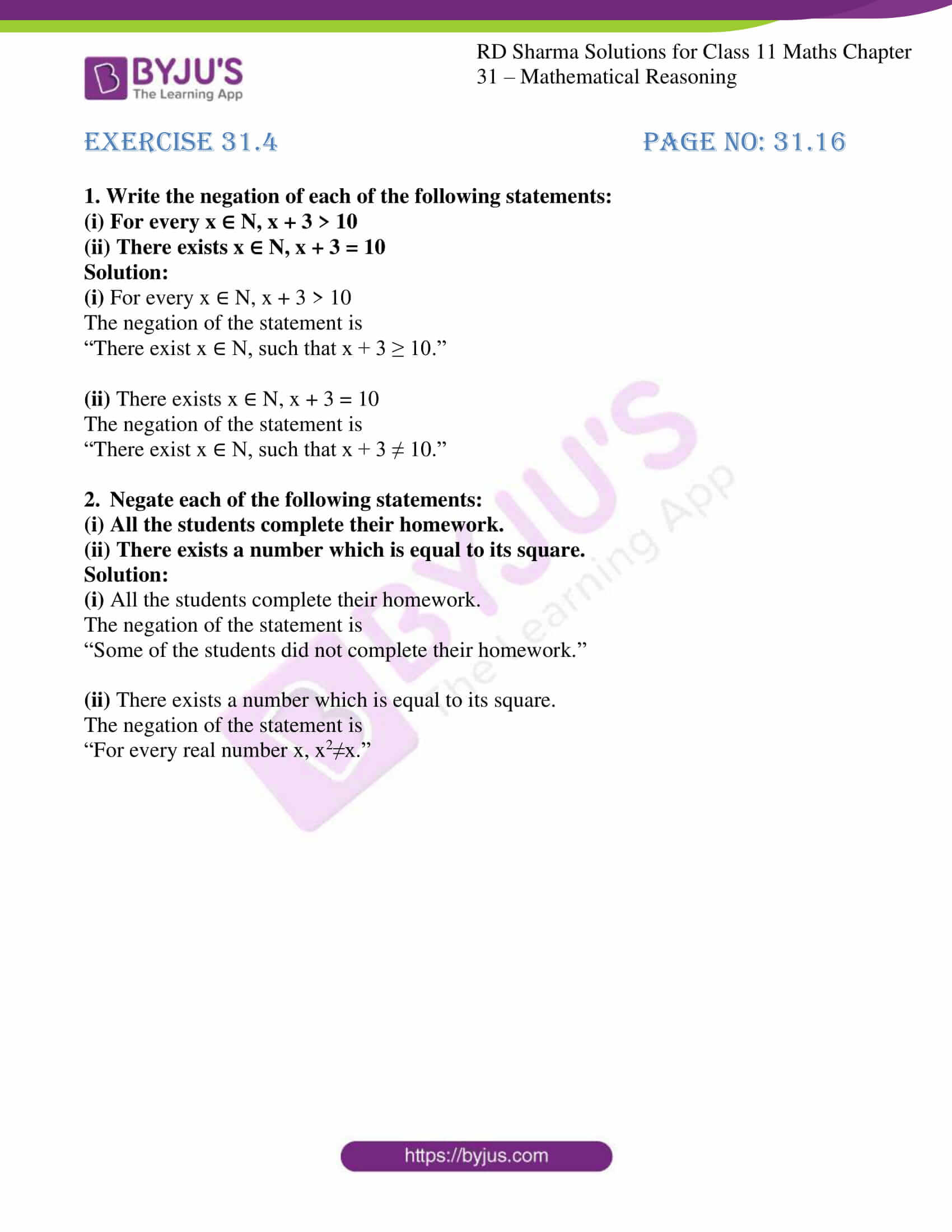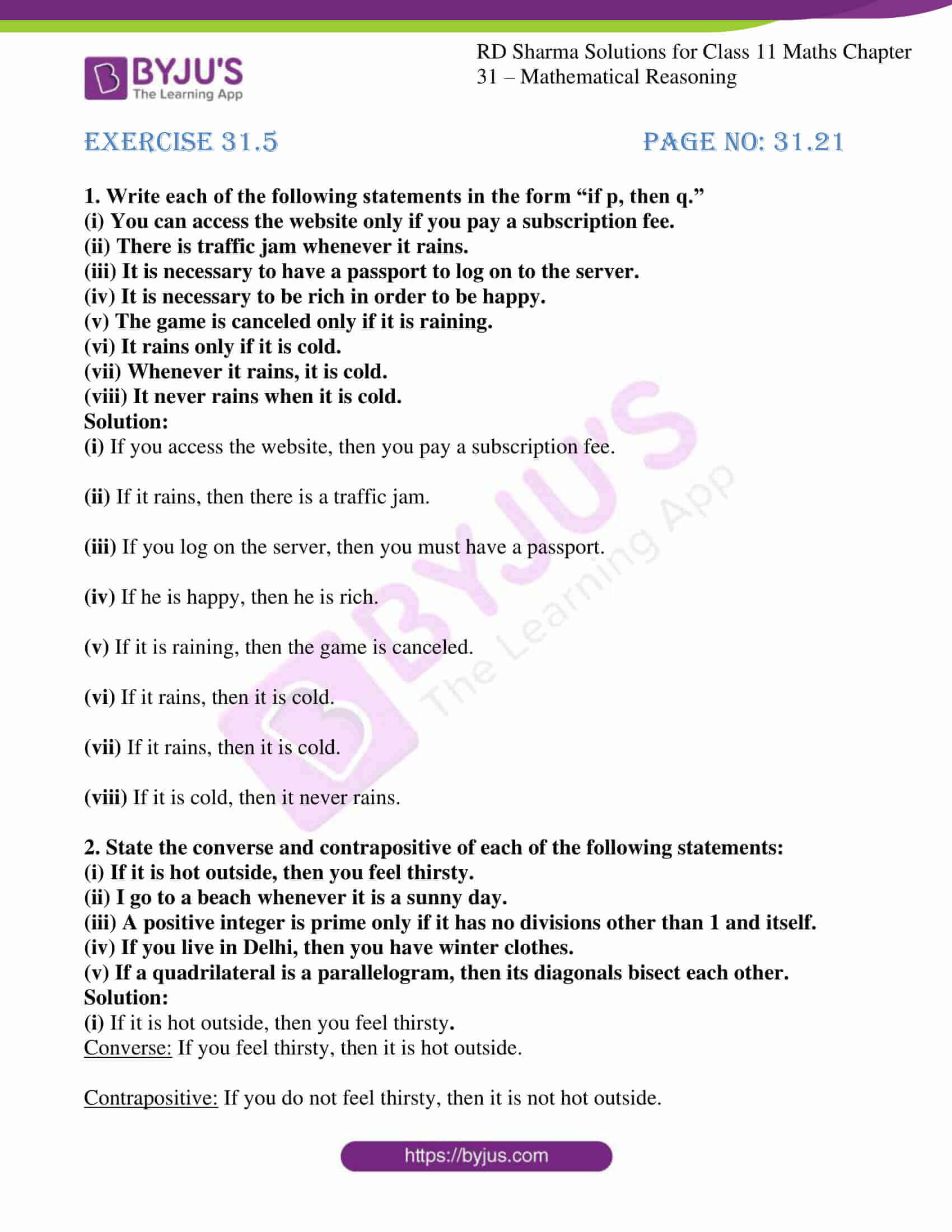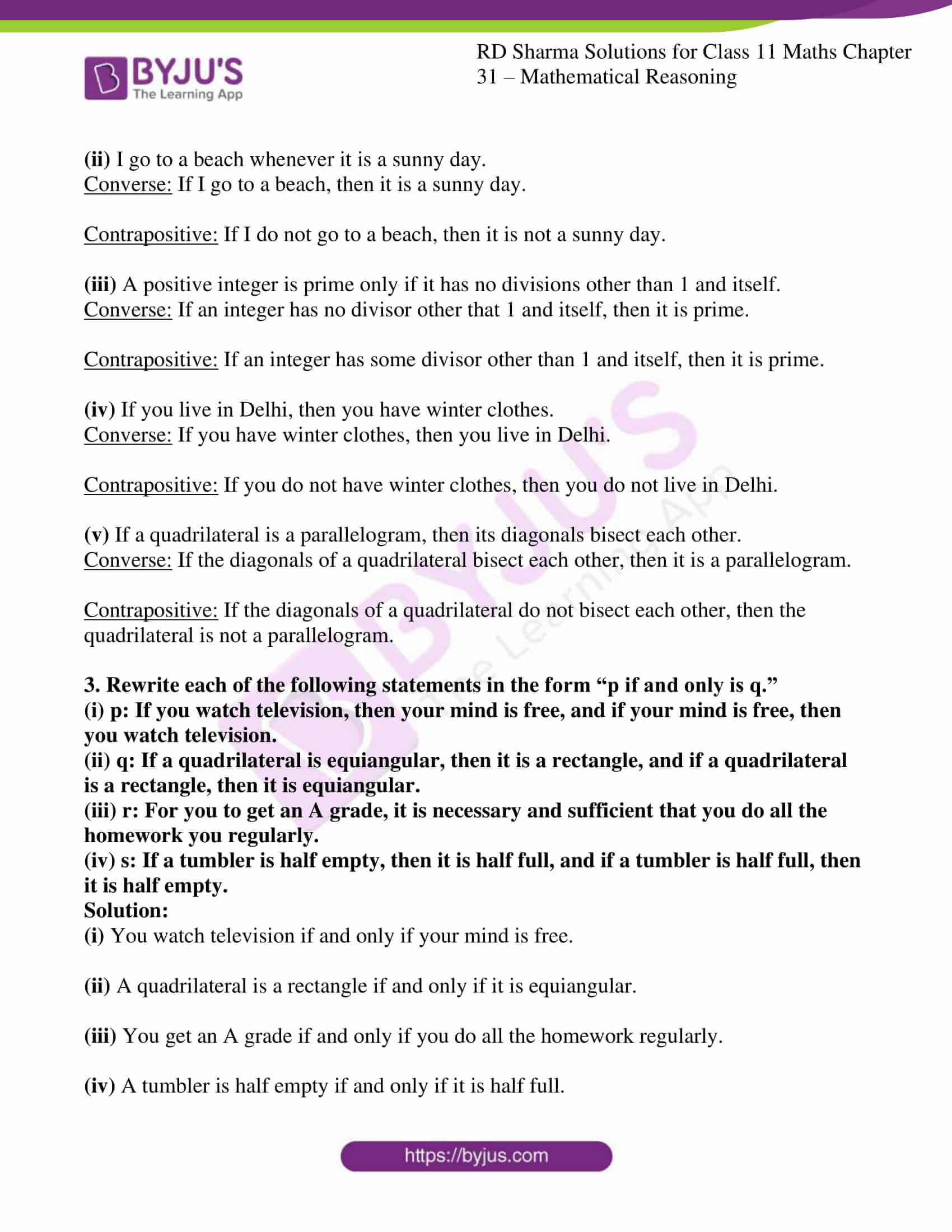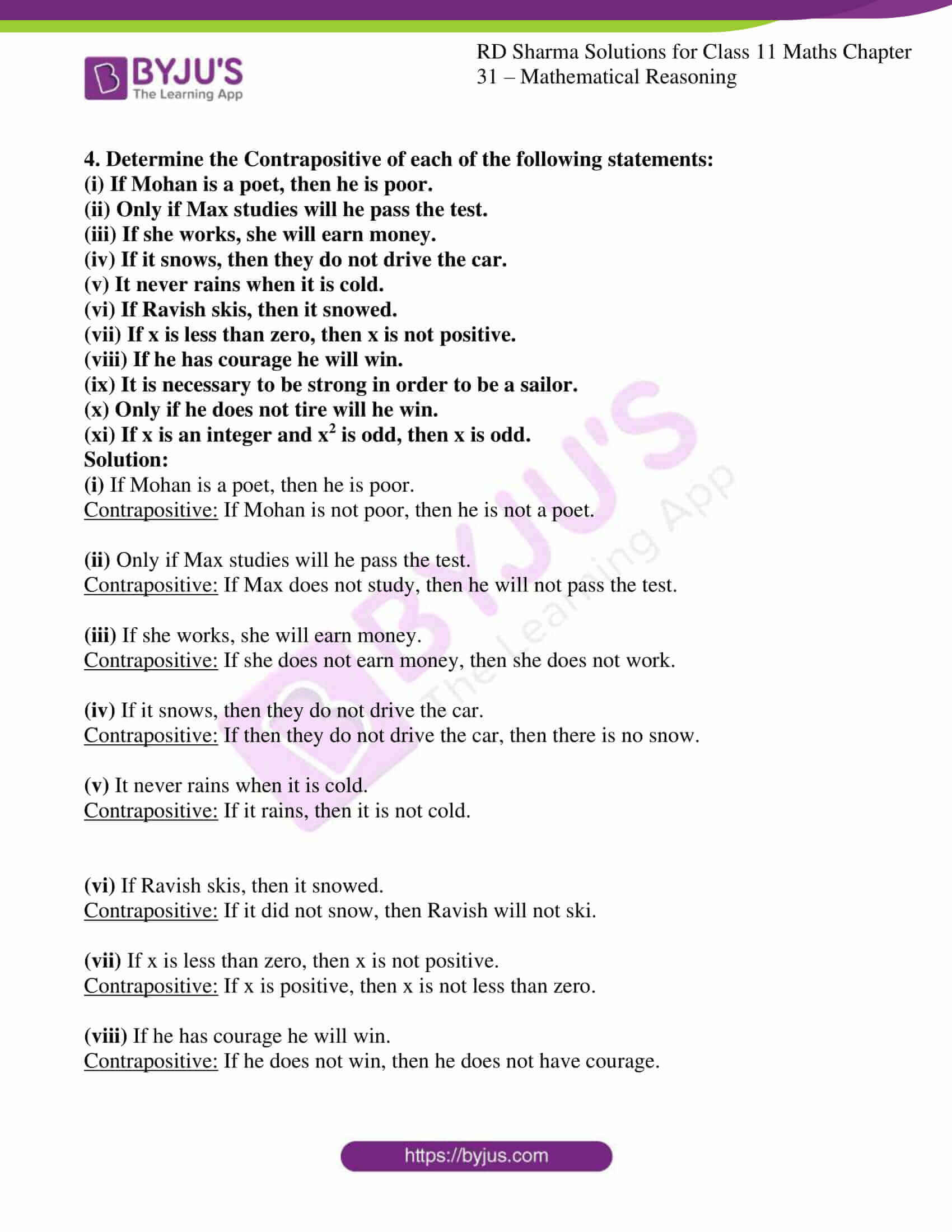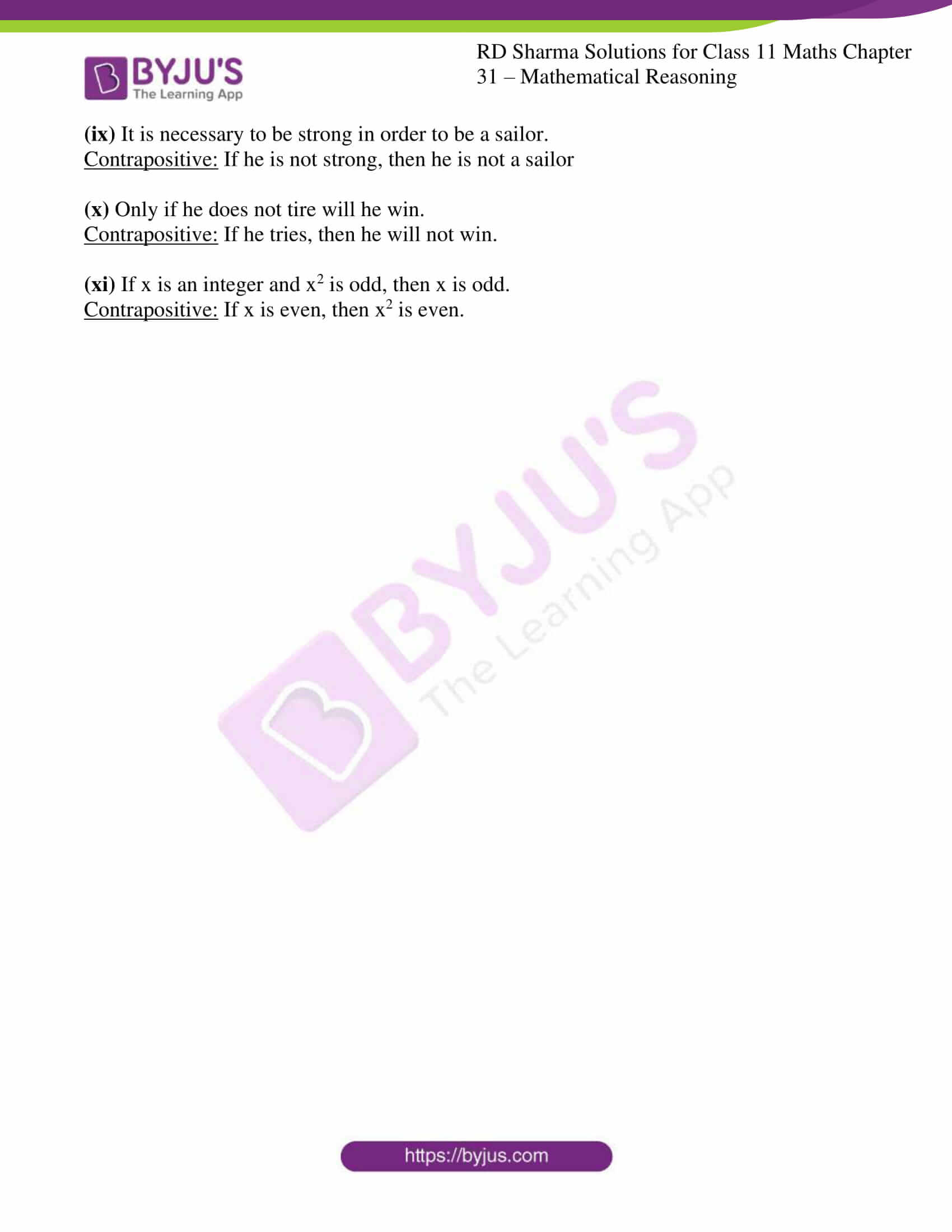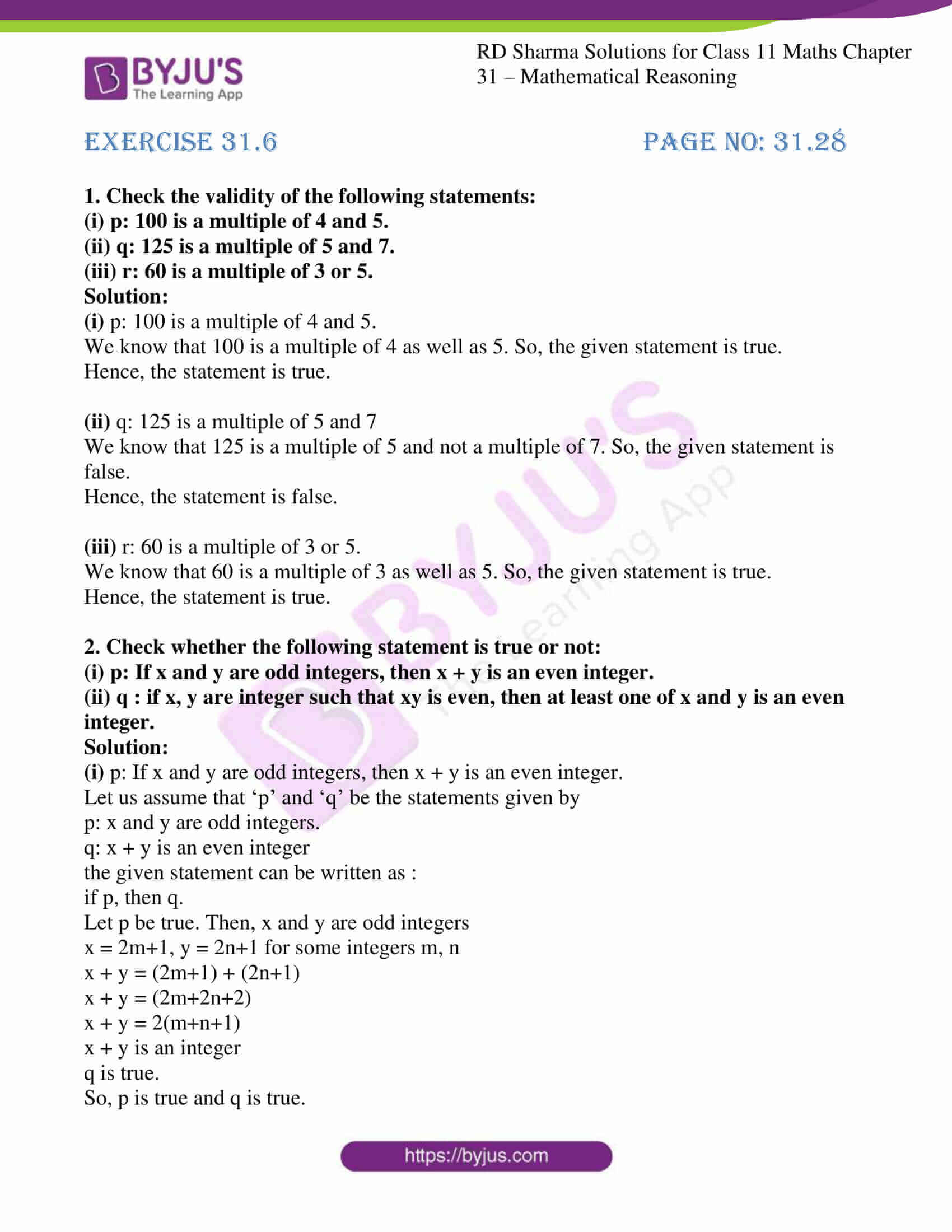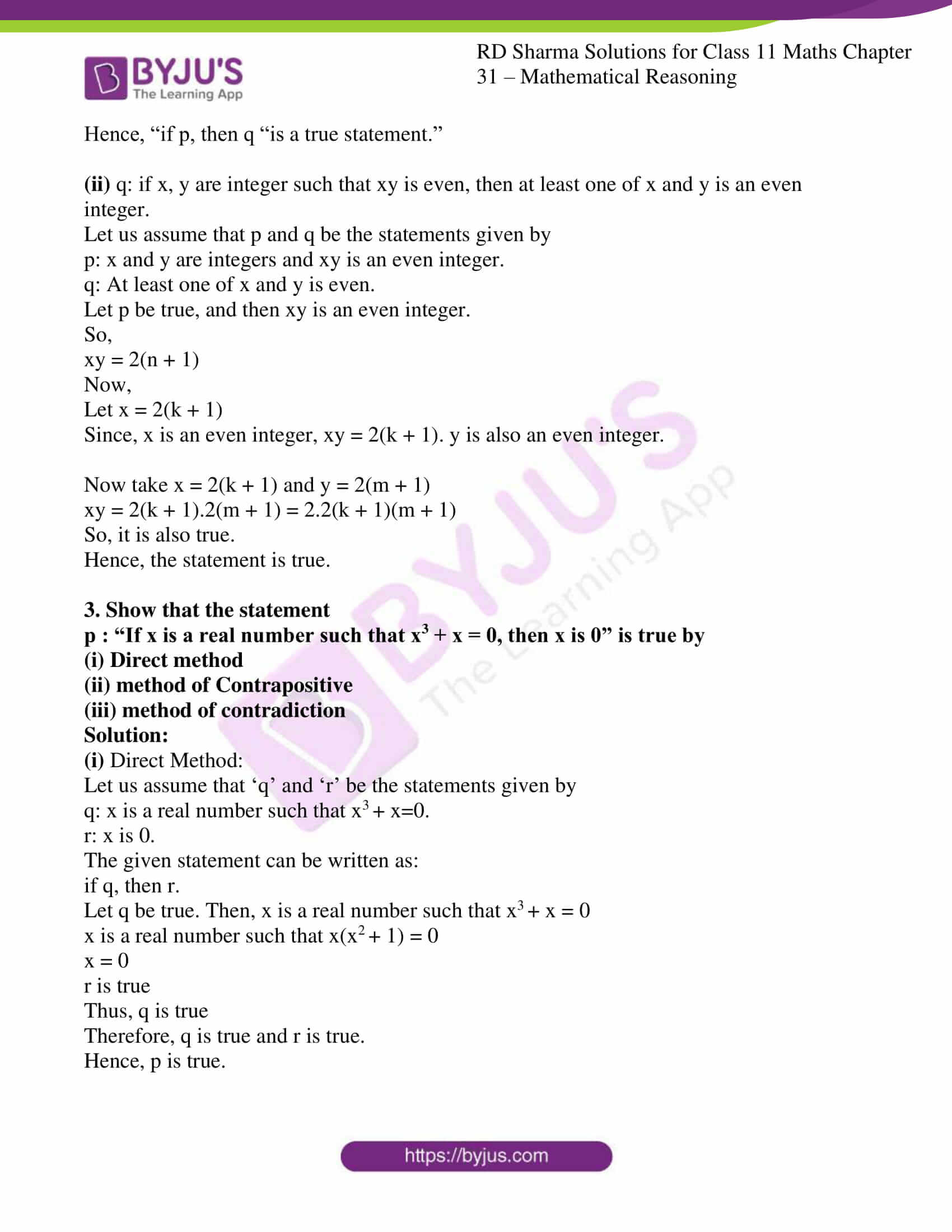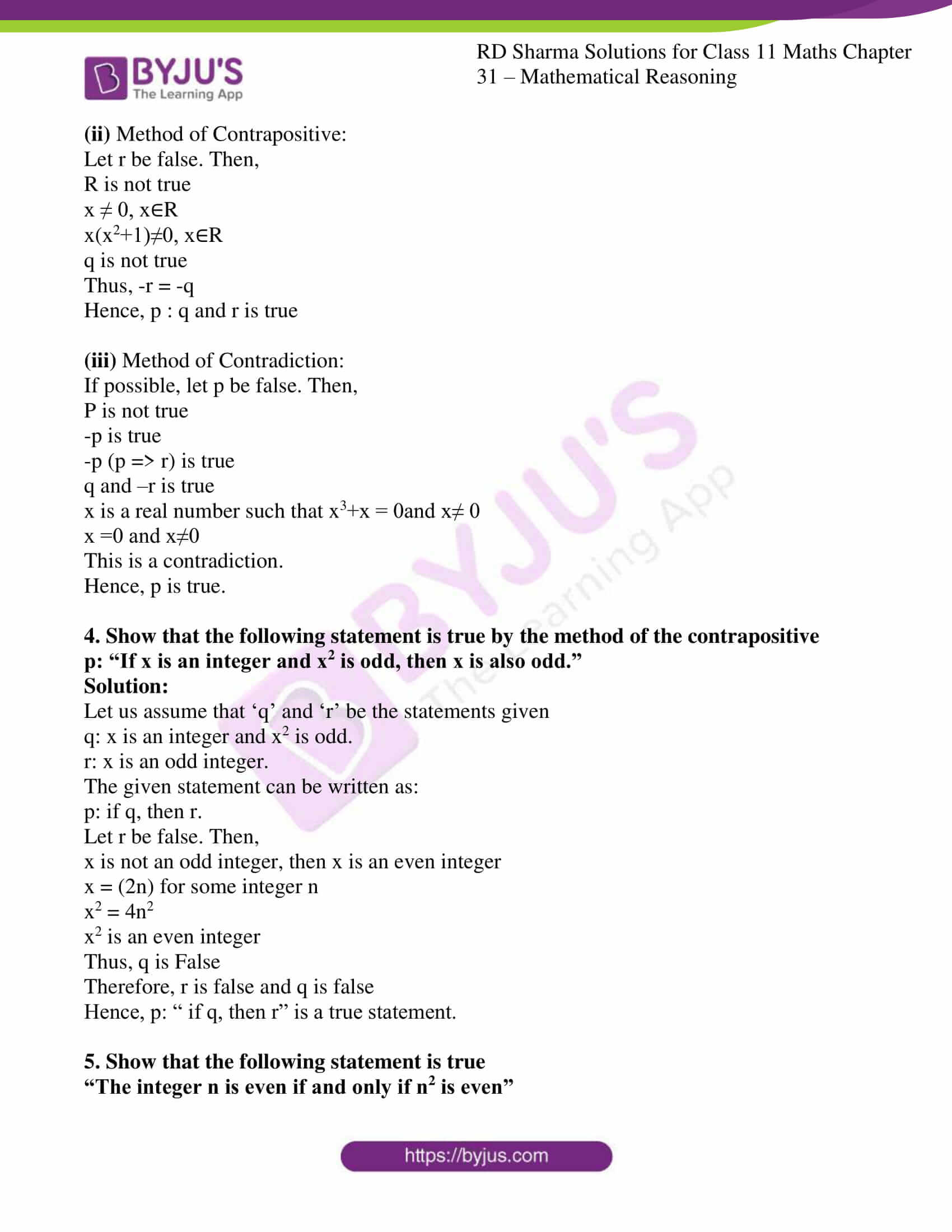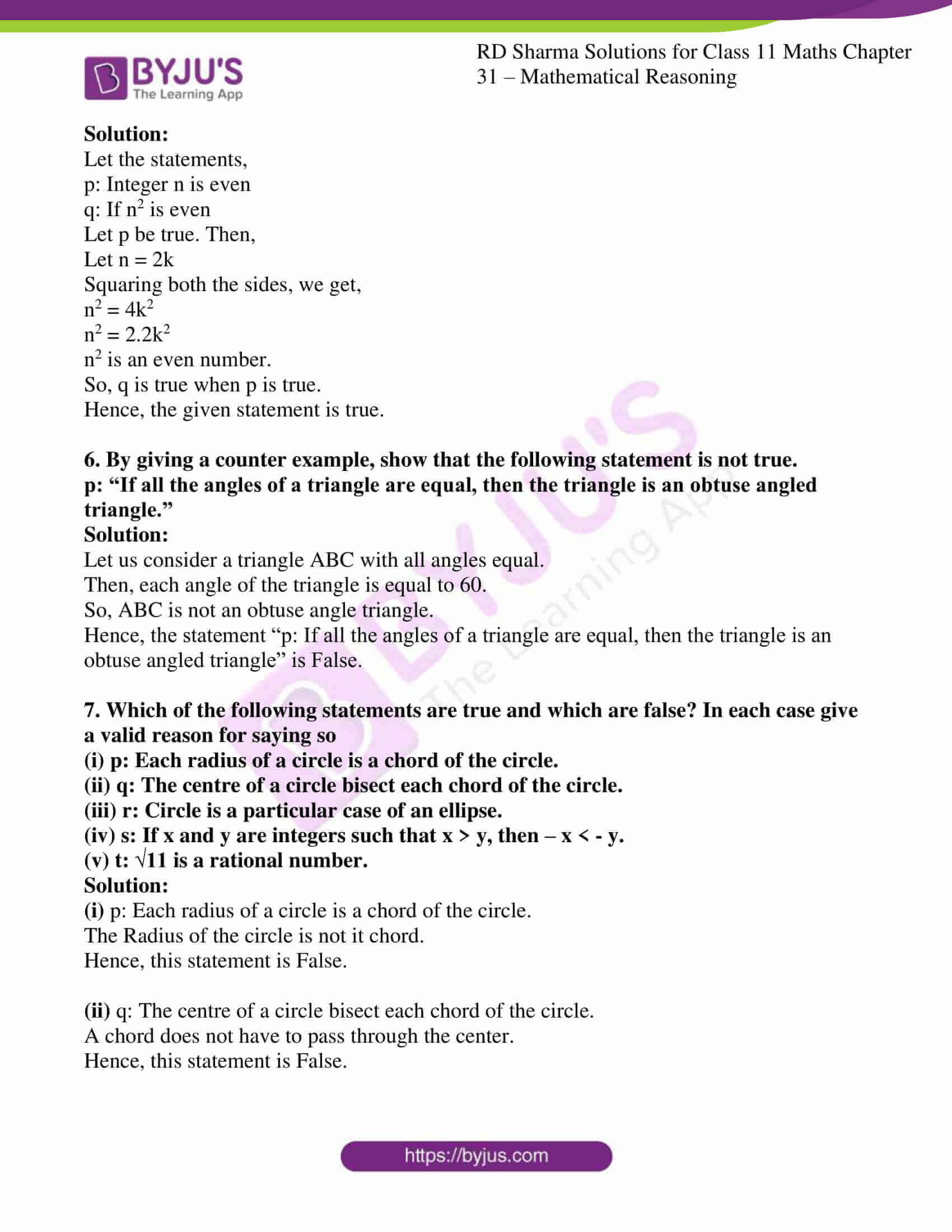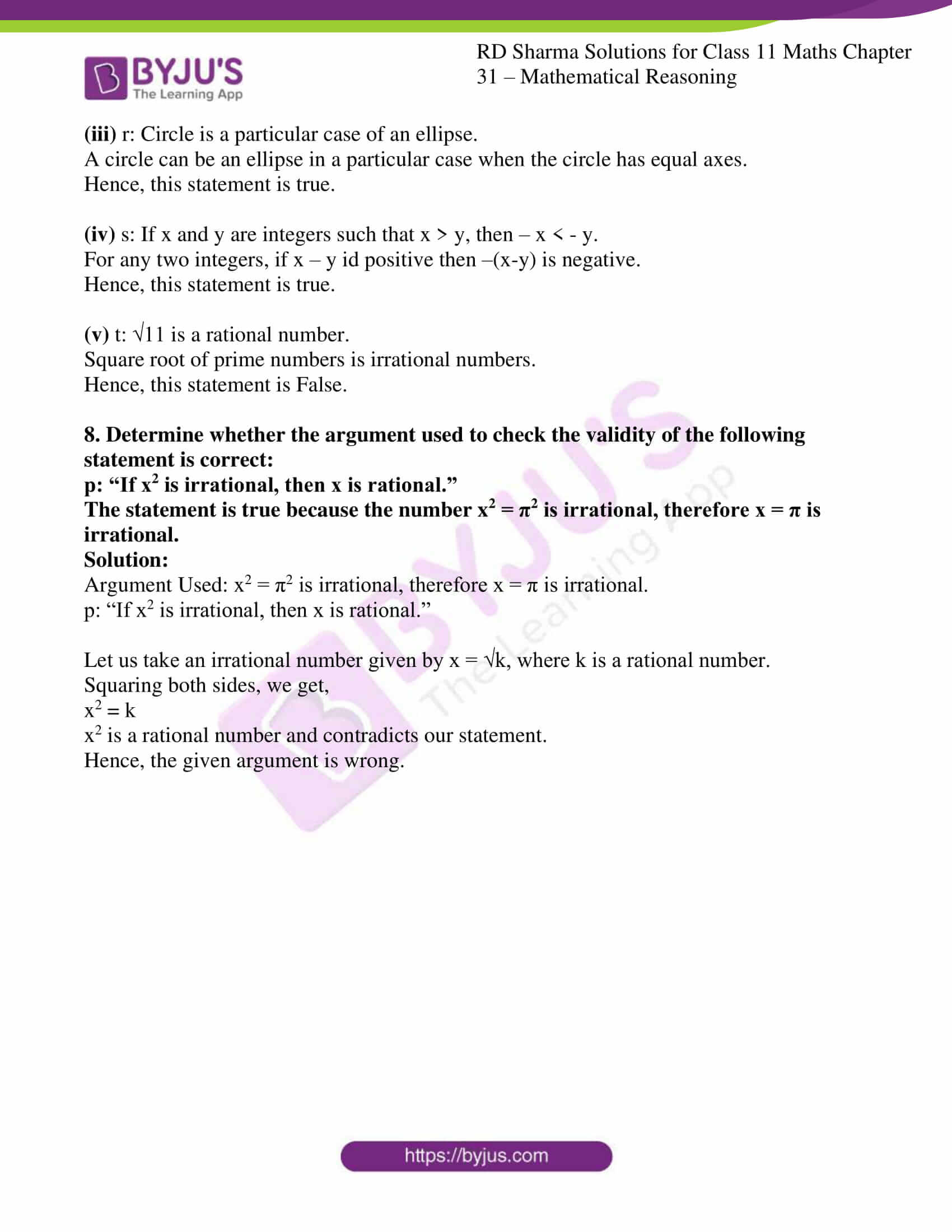### Access answers to RD Sharma Solutions for Class 11 Maths Chapter 31 – Mathematical Reasoning

#### EXERCISE 31.1 PAGE NO: 31.3

1. Find out which of the following sentences are statements and which are not. Justify your answer.
(i) Listen to me, Ravi!

(ii) Every set is a finite set.

(iii) Two non-empty sets have always a non-empty intersection.

(iv) The cat pussy is black.

(v) Are all circles round?

(vi) All triangles have three sides.

(vii) Every rhombus is a square.

(viii) x2 + 5|x| + 6 = 0 has no real roots.

(ix) This sentences is a statement.

(x) Is the earth round?

Solution:

(i) Listen to me, Ravi!

The sentence “Listen to me, Ravi! “Is an exclamatory sentence.

∴ It is not a statement.

(ii) Every set is a finite set.

This sentence is always false, because there are sets which are not finite.

∴ It is a statement.

(iii) Two non-empty sets have always a non-empty intersection.

This sentence is always false, because there are non-empty sets whose intersection is empty.

∴ It is a statement.

(iv) The cat pussy is black.

There are some cats which are black, and not black, So, the given sentence may or may not be true.

∴ It is not a statement.

(v) Are all circles round?

The given sentence is an interrogative sentence.

∴ It is not a statement.

(vi) All triangles have three sides.

The given sentence is a true declarative sentence.

∴ It is a true statement.

(vii) Every rhombus is a square.

This sentence is always false, because Rhombuses are not a square.

∴ It is a statement.

(viii) x2 + 5|x| + 6 = 0 has no real roots.

Firstly, let us solve the given expression.

If x>0,

x2+5|x|+6=0

x2+5x+6=0

x = -3 or x = -2

Since x>0, the equation has no roots.

If x<0,

x2+5|x|+6=0

x2 – 5x+6=0

Since x<0, the equation has no real roots.

∴ It is a statement.

(ix) This sentences is a statement.

The statement is not indicating the correct value, hence we can say that value contradicts the sense of the sentence.

∴ It is not a statement.

(x) Is the earth round?

The given sentence is an interrogative sentence.

∴ It is not a statement.

2. Give three examples of sentences which are not statements. Give reasons for the answers.

Solution:

a) “We won the match!”

The sentence “We won the match!” Is an exclamatory sentence.

∴ It is not a statement.

b) Can u get me cup of tea?

This sentence is an interrogative sentence.

∴ It is not a statement.

c) Please get me the book which is kept on the table.

This sentence is expressed either as a request or as a command, hence it is an imperative sentence.

∴ It is not a statement.

#### EXERCISE 31.2 PAGE NO: 31.6

1. Write the negation of the following statement:
(i) Bangalore is the capital of Karnataka.

(ii) It rained on July 4, 2005.

(iii) Ravish is honest.

(iv) The earth is round.

(v) The sun is cold.

Solution:

(i) Bangalore is the capital of Karnataka.

The negation of the statement is:

It is false that “Bangalore is the capital of Karnataka.”

Or

“Bangalore is not the capital of Karnataka.”

(ii) It rained on July 4, 2005.

The negation of the statement is:

It is false that “It rained on July 4, 2005”.

Or

“It did not rain on July 4, 2005”.

(iii) Ravish is honest.

The negation of the statement is:

It is false that “Ravish is honest.”

Or

“Ravish is not honest.”

(iv) The earth is round.

The negation of the statement is:

It is false that “The earth is round.”

Or

“The earth is not round.”

(v) The sun is cold.

The negation of the statement is:

It is false that “The sun is cold.”

Or

“The sun is not cold.”

2. (i) All birds sing.

(ii) Some even integers are prime.

(iii) There is a complex number which is not a real number.

(iv) I will not go to school.

(v) Both the diagonals of a rectangle have the same length.

(vi) All policemen are thieves.

Solution:

(i) All birds sing.

The negation of the statement is:

It is false that “All birds sing.”

Or

“All birds do not sing.”

(ii) Some even integers are prime.

The negation of the statement is:

It is false that “even integers are prime.”

Or

“Not every even integers is prime.”

(iii) There is a complex number which is not a real number.

The negation of the statement is:

It is false that “complex numbers are not a real number.”

Or

“All complex number are real numbers.”

(iv) I will not go to school.

The negation of the statement is:

“I will go to school.”

(v) Both the diagonals of a rectangle have the same length.

The negation of the statement is:

“There is at least one rectangle whose both diagonals do not have the same length.”

(vi) All policemen are thieves.

The negation of the statement is:

“No policemen are thief”.

3. Are the following pairs of statements are a negation of each other:
(i) The number x is not a rational number.
The number x is not an irrational number.
(ii) The number x is not a rational number.
The number x is an irrational number.

Solution:

(i) The number x is not a rational number.

“The number x is an irrational number.”

Since, the statement “The number x is not a rational number.” Is a negation of the first statement.

(ii) The number x is not a rational number.

“The number x is an irrational number.”

Since, the statement “The number x is a rational number.” Is not a negation of the first statement.

4. Write the negation of the following statements:

(i) p: For every positive real number x, the number (x – 1) is also positive.

(ii) q: For every real number x, either x > 1 or x < 1.

(iii) r: There exists a number x such that 0 < x < 1.

Solution:

(i) p : For every positive real number x, the number (x – 1) is also positive.

The negation of the statement:

p: For every positive real number x, the number (x – 1) is also positive.

is

~p: There exists a positive real number x, such that the number (x – 1) is not positive.

(ii) q: For every real number x, either x > 1 or x < 1.

The negation of the statement:

q: For every real number x, either x > 1 or x < 1.

is

~q: There exists a real number such that neither x>1 or x<1.

(iii) r: There exists a number x such that 0 < x < 1.

The negation of the statement:

r: There exists a number x such that 0 < x < 1.

is

~r: For every real number x, either x ≤ 0 or x ≥ 1.

5. Check whether the following pair of statements is a negation of each other. Give reasons for your answer.

(i) a + b = b + a is true for every real number a and b.
(ii) There exist real numbers a and b for which a + b = b + a.

Solution:

The negation of the statement:

p: a + b = b + a is a true for every real number a and b.

is

~p: There exist real numbers are ‘a’ and ‘b’ for which a+b ≠ b+a.

So, the given statement is not the negation of the first statement.

#### EXERCISE 31.3 PAGE NO: 31.14

1. Find the component statements of the following compound statements:
(i) The sky is blue, and the grass is green.
(ii) The earth is round, or the sun is cold.
(iii) All rational numbers are real, and all real numbers are complex.
(iv) 25 is a multiple of 5 and 8.

Solution:

(i) The components of the compound statement are:

P: The sky is blue.

Q: The grass is green.

(ii) The components of the compound statement are:

P: The earth is round.

Q: The sun is cold.

(iii) The components of the compound statement are:

P: All rational number is real.

Q: All real number are complex.

(iv) The components of the compound statement are:

P: 25 is multiple of 5.

Q: 25 is multiple of 8.

2. For each of the following statements, determine whether an inclusive “OR” o exclusive “OR” is used. Give reasons for your answer.
(i) Students can take Hindi or Sanskrit as their third language.
(ii) To entry a country, you need a passport or a voter registration card.
(iii) A lady gives birth to a baby boy or a baby girl.
(iv) To apply for a driving license, you should have a ration card or a passport.

Solution:

(i) In the given statement “Students can take Hindi or Sanskrit as their third language.”

An exclusive “OR” is used because a student cannot take both Hindi and Sanskrit as the third language.

(ii) In the given statement “To entry a country, you need a passport or a voter registration card.”

An inclusive “OR” is used because a person can have both a passport and a voter registration card to enter a country.

(iii) In the given statement “A lady gives birth to a baby boy or a baby girl.”

An exclusive “OR” is used because a lady cannot give birth to a baby who is both a boy and a girl.

(iv) In the given statement “To apply for a driving license, you should have a ration card or a passport.”

An inclusive “OR” is used because a person can have both a ration card and passport to apply for a driving license.

3. Write the component statements of the following compound statements and check whether the compound statement is true or false:
(i) To enter into a public library children need an identification card from the school or a letter from the school authorities.
(ii) All rational numbers are real and all real numbers are not complex.
(iii) Square of an integer is positive or negative.
(iv) x = 2 and x = 3 are the roots of the equation 3x2 – x – 10 = 0.
(v) The sand heats up quickly in the sun and does not cool down fast at night.

Solution:

(i) The components of the compound statement are:

P: To get into a public library children need an identity card.

Q: To get into a public library children need a letter from the school authorities.

Both P and Q are true.

Hence, the compound statement is true.

(ii) The components of the compound statement are:

P: All rational number is real.

Q: All real numbers are not complex.

P is true and Q is false then P and Q both are False.

Hence, the compound statement is False

(iii) The components of the compound statement are:

P: Square of an integer is positive.

Q: Square of an integer is negative.

Both P and Q are true.

Hence, the compound statement is True.

(iv) The components of the compound statement are:

P: x=2 is a root of the equation 3x2 – x – 10 = 0

Q: x = 3 is a root of the equation 3x2 – x – 10 = 0

P is true, but Q is false then P and Q both are False.

Hence, the compound statement is False.

(v) The components of the compound statement are:

P: The sand heats up quickly in the sun.

Q: The sand does not cool down fast at night.

P is false and Q is also false then P and Q both are False.

Hence, the compound statement is False.

4. Determine whether the following compound statements are true or false:
(i) Delhi is in India and 2 + 2 = 4
(ii) Delhi is in England and 2 + 2 = 4
(iii) Delhi is in India and 2 + 2 = 5
(iv) Delhi is in England and 2 + 2 = 5

Solution:

(i) The components of the compound statement are:

P: Delhi is in India.

Q: 2 + 2 = 4

Both P and Q are true.

Hence, the compound statement is True.

(ii) The components of the compound statement are:

P: Delhi is in England.

Q: 2 + 2 = 4

P is false, and q is true. So, both P and Q are false.

Hence, the compound statement is False.

(iii) The components of the compound statement are:

P: Delhi is in India.

Q: 2 + 2 = 5

P is true, and q is false. So, both P and Q are false.

Hence, the compound statement is False.

(iv) The components of the compound statement are:

P: Delhi is in England.

Q: 2 + 2 = 5

Both P and Q are false.

Hence, the compound statement is False.

#### EXERCISE 31.4 PAGE NO: 31.16

1. Write the negation of each of the following statements:
(i) For every x ∈ N, x + 3 > 10
(ii) There exists x ∈ N, x + 3 = 10

Solution:

(i) For every x ∈ N, x + 3 > 10

The negation of the statement is

“There exist x ∈ N, such that x + 3 ≥ 10.”

(ii) There exists x ∈ N, x + 3 = 10

The negation of the statement is

“There exist x ∈ N, such that x + 3 ≠ 10.”

2. Negate each of the following statements:
(i) All the students complete their homework.
(ii) There exists a number which is equal to its square.

Solution:

(i) All the students complete their homework.

The negation of the statement is

“Some of the students did not complete their homework.”

(ii) There exists a number which is equal to its square.

The negation of the statement is

“For every real number x, x2≠x.”

#### EXERCISE 31.5 PAGE NO: 31.21

1. Write each of the following statements in the form “if p, then q.”
(i) You can access the website only if you pay a subscription fee.
(ii) There is traffic jam whenever it rains.
(iii) It is necessary to have a passport to log on to the server.
(iv) It is necessary to be rich in order to be happy.
(v) The game is canceled only if it is raining.
(vi) It rains only if it is cold.
(vii) Whenever it rains, it is cold.
(viii) It never rains when it is cold.

Solution:

(i) If you access the website, then you pay a subscription fee.

(ii) If it rains, then there is a traffic jam.

(iii) If you log on the server, then you must have a passport.

(iv) If he is happy, then he is rich.

(v) If it is raining, then the game is canceled.

(vi) If it rains, then it is cold.

(vii) If it rains, then it is cold.

(viii) If it is cold, then it never rains.

2. State the converse and contrapositive of each of the following statements:
(i) If it is hot outside, then you feel thirsty.

(ii) I go to a beach whenever it is a sunny day.

(iii) A positive integer is prime only if it has no divisions other than 1 and itself.

(iv) If you live in Delhi, then you have winter clothes.

(v) If a quadrilateral is a parallelogram, then its diagonals bisect each other.

Solution:

(i) If it is hot outside, then you feel thirsty.

Converse: If you feel thirsty, then it is hot outside.

Contrapositive: If you do not feel thirsty, then it is not hot outside.

(ii) I go to a beach whenever it is a sunny day.

Converse: If I go to a beach, then it is a sunny day.

Contrapositive: If I do not go to a beach, then it is not a sunny day.

(iii) A positive integer is prime only if it has no divisions other than 1 and itself.

Converse: If an integer has no divisor other that 1 and itself, then it is prime.

Contrapositive: If an integer has some divisor other than 1 and itself, then it is prime.

(iv) If you live in Delhi, then you have winter clothes.

Converse: If you have winter clothes, then you live in Delhi.

Contrapositive: If you do not have winter clothes, then you do not live in Delhi.

(v) If a quadrilateral is a parallelogram, then its diagonals bisect each other.

Converse: If the diagonals of a quadrilateral bisect each other, then it is a parallelogram.

Contrapositive: If the diagonals of a quadrilateral do not bisect each other, then the quadrilateral is not a parallelogram.

3. Rewrite each of the following statements in the form “p if and only is q.”
(i) p: If you watch television, then your mind is free, and if your mind is free, then you watch television.
(ii) q: If a quadrilateral is equiangular, then it is a rectangle, and if a quadrilateral is a rectangle, then it is equiangular.
(iii) r: For you to get an A grade, it is necessary and sufficient that you do all the homework you regularly.
(iv) s: If a tumbler is half empty, then it is half full, and if a tumbler is half full, then it is half empty.

Solution:

(i) You watch television if and only if your mind is free.

(ii) A quadrilateral is a rectangle if and only if it is equiangular.

(iii) You get an A grade if and only if you do all the homework regularly.

(iv) A tumbler is half empty if and only if it is half full.

4. Determine the Contrapositive of each of the following statements:
(i) If Mohan is a poet, then he is poor.
(ii) Only if Max studies will he pass the test.
(iii) If she works, she will earn money.
(iv) If it snows, then they do not drive the car.
(v) It never rains when it is cold.
(vi) If Ravish skis, then it snowed.
(vii) If x is less than zero, then x is not positive.
(viii) If he has courage he will win.
(ix) It is necessary to be strong in order to be a sailor.
(x) Only if he does not tire will he win.
(xi) If x is an integer and x2 is odd, then x is odd.

Solution:

(i) If Mohan is a poet, then he is poor.

Contrapositive: If Mohan is not poor, then he is not a poet.

(ii) Only if Max studies will he pass the test.

Contrapositive: If Max does not study, then he will not pass the test.

(iii) If she works, she will earn money.

Contrapositive: If she does not earn money, then she does not work.

(iv) If it snows, then they do not drive the car.

Contrapositive: If then they do not drive the car, then there is no snow.

(v) It never rains when it is cold.

Contrapositive: If it rains, then it is not cold.

(vi) If Ravish skis, then it snowed.

Contrapositive: If it did not snow, then Ravish will not ski.

(vii) If x is less than zero, then x is not positive.

Contrapositive: If x is positive, then x is not less than zero.

(viii) If he has courage he will win.

Contrapositive: If he does not win, then he does not have courage.

(ix) It is necessary to be strong in order to be a sailor.

Contrapositive: If he is not strong, then he is not a sailor

(x) Only if he does not tire will he win.

Contrapositive: If he tries, then he will not win.

(xi) If x is an integer and x2 is odd, then x is odd.

Contrapositive: If x is even, then x2 is even.

#### EXERCISE 31.6 PAGE NO: 31.28

1. Check the validity of the following statements:
(i) p: 100 is a multiple of 4 and 5.
(ii) q: 125 is a multiple of 5 and 7.
(iii) r: 60 is a multiple of 3 or 5.

Solution:

(i) p: 100 is a multiple of 4 and 5.

We know that 100 is a multiple of 4 as well as 5. So, the given statement is true.

Hence, the statement is true.

(ii) q: 125 is a multiple of 5 and 7

We know that 125 is a multiple of 5 and not a multiple of 7. So, the given statement is false.

Hence, the statement is false.

(iii) r: 60 is a multiple of 3 or 5.

We know that 60 is a multiple of 3 as well as 5. So, the given statement is true.

Hence, the statement is true.

2. Check whether the following statement is true or not:
(i) p: If x and y are odd integers, then x + y is an even integer.

(ii) q : if x, y are integer such that xy is even, then at least one of x and y is an even integer.

Solution:

(i) p: If x and y are odd integers, then x + y is an even integer.

Let us assume that ‘p’ and ‘q’ be the statements given by

p: x and y are odd integers.

q: x + y is an even integer

the given statement can be written as :

if p, then q.

Let p be true. Then, x and y are odd integers

x = 2m+1, y = 2n+1 for some integers m, n

x + y = (2m+1) + (2n+1)

x + y = (2m+2n+2)

x + y = 2(m+n+1)

x + y is an integer

q is true.

So, p is true and q is true.

Hence, “if p, then q “is a true statement.”

(ii) q: if x, y are integer such that xy is even, then at least one of x and y is an even integer.

Let us assume that p and q be the statements given by

p: x and y are integers and xy is an even integer.

q: At least one of x and y is even.

Let p be true, and then xy is an even integer.

So,

xy = 2(n + 1)

Now,

Let x = 2(k + 1)

Since, x is an even integer, xy = 2(k + 1). y is also an even integer.

Now take x = 2(k + 1) and y = 2(m + 1)

xy = 2(k + 1).2(m + 1) = 2.2(k + 1)(m + 1)

So, it is also true.

Hence, the statement is true.

3. Show that the statement
p : “If x is a real number such that x3 + x = 0, then x is 0” is true by
(i) Direct method
(ii) method of Contrapositive

Solution:

(i) Direct Method:

Let us assume that ‘q’ and ‘r’ be the statements given by

q: x is a real number such that x3 + x=0.

r: x is 0.

The given statement can be written as:

if q, then r.

Let q be true. Then, x is a real number such that x3 + x = 0

x is a real number such that x(x2 + 1) = 0

x = 0

r is true

Thus, q is true

Therefore, q is true and r is true.

Hence, p is true.

(ii) Method of Contrapositive:

Let r be false. Then,

R is not true

x ≠ 0, x∈R

x(x2+1)≠0, x∈R

q is not true

Thus, -r = -q

Hence, p : q and r is true

If possible, let p be false. Then,

P is not true

-p is true

-p (p => r) is true

q and –r is true

x is a real number such that x3+x = 0and x≠ 0

x =0 and x≠0

Hence, p is true.

4. Show that the following statement is true by the method of the contrapositive
p: “If x is an integer and x2 is odd, then x is also odd.”

Solution:

Let us assume that ‘q’ and ‘r’ be the statements given

q: x is an integer and x2 is odd.

r: x is an odd integer.

The given statement can be written as:

p: if q, then r.

Let r be false. Then,

x is not an odd integer, then x is an even integer

x = (2n) for some integer n

x2 = 4n2

x2 is an even integer

Thus, q is False

Therefore, r is false and q is false

Hence, p: “ if q, then r” is a true statement.

5. Show that the following statement is true
“The integer n is even if and only if n2 is even”

Solution:

Let the statements,

p: Integer n is even

q: If n2 is even

Let p be true. Then,

Let n = 2k

Squaring both the sides, we get,

n2 = 4k2

n2 = 2.2k2

n2 is an even number.

So, q is true when p is true.

Hence, the given statement is true.

6. By giving a counter example, show that the following statement is not true.
p: “If all the angles of a triangle are equal, then the triangle is an obtuse angled triangle.”

Solution:

Let us consider a triangle ABC with all angles equal.

Then, each angle of the triangle is equal to 60.

So, ABC is not an obtuse angle triangle.

Hence, the statement “p: If all the angles of a triangle are equal, then the triangle is an obtuse angled triangle” is False.

7. Which of the following statements are true and which are false? In each case give a valid reason for saying so
(i) p: Each radius of a circle is a chord of the circle.
(ii) q: The centre of a circle bisect each chord of the circle.
(iii) r: Circle is a particular case of an ellipse.
(iv) s: If x and y are integers such that x > y, then – x < – y.
(v) t: √11 is a rational number.

Solution:

(i) p: Each radius of a circle is a chord of the circle.

The Radius of the circle is not it chord.

Hence, this statement is False.

(ii) q: The centre of a circle bisect each chord of the circle.

A chord does not have to pass through the center.

Hence, this statement is False.

(iii) r: Circle is a particular case of an ellipse.

A circle can be an ellipse in a particular case when the circle has equal axes.

Hence, this statement is true.

(iv) s: If x and y are integers such that x > y, then – x < – y.

For any two integers, if x – y id positive then –(x-y) is negative.

Hence, this statement is true.

(v) t: √11 is a rational number.

Square root of prime numbers is irrational numbers.

Hence, this statement is False.

8. Determine whether the argument used to check the validity of the following statement is correct:
p: “If x2 is irrational, then x is rational.”
The statement is true because the number x2 = π2 is irrational, therefore x = π is irrational.

Solution:

Argument Used: x2 = π2 is irrational, therefore x = π is irrational.

p: “If x2 is irrational, then x is rational.”

Let us take an irrational number given by x = √k, where k is a rational number.

Squaring both sides, we get,

x2 = k

x2 is a rational number and contradicts our statement.

Hence, the given argument is wrong.

### Also, access exercises of RD Sharma Solutions for Class 11 Maths Chapter 31 – Mathematical Reasoning

Exercise 31.1 Solutions

Exercise 31.2 Solutions

Exercise 31.3 Solutions

Exercise 31.4 Solutions

Exercise 31.5 Solutions

Exercise 31.6 Solutions

## Frequently Asked Questions on RD Sharma Solutions for Class 11 Maths Chapter 31

### What are the key points of using the RD Sharma Solutions for Class 11 Maths Chapter 31?

The key points of using the RD Sharma Solutions for Class 11 Maths Chapter 31 are:
1. It provides solutions for all the textbook questions.
2. Individual subject matter experts have prepared the solutions as per the CBSE guidelines and marking schemes.
3. BYJU’S RD Sharma Solutions are the most reliable study guides that students can confidently rely on for exam preparations.
4. Improves conceptual learning and analytical reasoning among students.

### How can students prepare for the annual exam using the RD Sharma Solutions for Class 11 Maths Chapter 31?

Before the annual exam, students have to learn the chapter thoroughly and revise them regularly to attain a strong grip over the key concepts. The RD Sharma Solutions developed by expert tutors at BYJU’S is an apt resource for exam preparations and to score high marks in the exam. It’s recommended that students refer to these solutions while attempting the questions to comprehend the method of answering as per the latest CBSE guidelines. Also, students must read thoroughly the syllabus for the academic year before the exam to understand the marks weightage for the chapter.

### Can the RD Sharma Solutions for Class 11 Maths Chapter 31 be considered as the best study material for exam preparations?

We at BYJU’S have provided solutions to all the textbook questions based on the latest CBSE guidelines. The solutions are compiled in PDFs so that students can download them for free and refer to it anywhere and anytime. All the solutions are framed by individual subject matter experts to help students ace the exam. The solutions contain detailed explanations in simple steps to boost the confidence of students in answering the difficult and long-answer type questions efficiently.